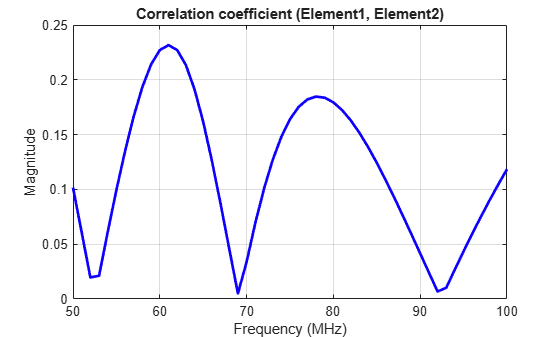# correlation

Correlation coefficient between two antennas in array

## Syntax

``correlation(array,frequency,elem1,elem2,z0)``
``rho = correlation(array,frequency,elem1,elem2,z0)``

## Description

example

````correlation(array,frequency,elem1,elem2,z0)` calculates and plots the correlation coefficient between two antenna elements, `elem1` and `elem2` of an array. The correlation values are calculated for a specified frequency and impedance and for a specified impedance `z0`.```

example

````rho = correlation(array,frequency,elem1,elem2,z0)` returns the correlation coefficient between two antenna elements, `elem1` and `elem2` of an array.```

## Examples

collapse all

Plot the correlation between 1 and 2 antenna elements in a default linear array over a frequency range of 50MHz to 100MHz.

```h = linearArray; correlation (h,50e6:1e6:100e6,1,2);```Calculate correlation coefficient of default rectangular array at a frequency range of 50MHz to 100MHz.

```h = rectangularArray; rho = correlation (h, 50e6:1e6:100e6, 1, 2)```
```rho = 51×1 0.1417 0.1123 0.0826 0.0524 0.0215 0.0102 0.0429 0.0763 0.1094 0.1409 ⋮ ```

## Input Arguments

collapse all

Array to calculate the correlation between its elements, specified as an array object from array catalog, `customArrayMesh` object or `customArrayGeometry` object.

Example: ```linearArray(Element=[dipole dipole(Length=1.5)])```

Frequency range to calculate correlation, specified as a scalar in Hz. for a single analysis frequency or a vector in Hz. for multiple analysis frequencies.

Example: 50e6:1e6:100e6

Data Types: `double`

Antenna elements in an array to calculate the correlation, specified as scalars represented by their element number in the array.

Example: `1,2`

Reference impedance, specified as a scalar in ohms.

Example: 70

Data Types: `double`

## Output Arguments

collapse all

Correlation coefficient between two antenna elements of an array, returned as a scalar for a single analysis frequency or a vector for multiple analysis frequencies.

Data Types: `double`

 S. Blanch, J. Romeu, and I. Corbella. Exact representation of antenna system diversity performance from input parameter description. Electron. Lett., vol. 39, pp. 705-707, May 2003.